Your skills with simultaneous equations are required to cost tins of paint in this quiz.

# Simultaneous Equations 2

This Math quiz is called 'Simultaneous Equations 2' and it has been written by teachers to help you if you are studying the subject at middle school. Playing educational quizzes is a fabulous way to learn if you are in the 6th, 7th or 8th grade - aged 11 to 14.

It costs only \$12.50 per month to play this quiz and over 3,500 others that help you with your school work. You can subscribe on the page at Join Us

This quiz on Simultaneous Equations builds on the previous quiz, have you tried that one yet? Make sure you have mastered the basics of the elimination and substitution methods of solving a system of linear equations before tackling this quiz, which includes quadratics!

When solving simultaneous equations, particularly complicated ones that include a quadratic, it’s always a good idea to consider what the equations look like on a graph. You should recall that the point, or points, where two lines (or curves) intersect is a solution to the system of simultaneous equations. If you can sketch out the curve and the straight line, it will give you an indication of how many solutions there are, and their approximate position on the grid.

When both the equations you are solving simultaneously are linear, you have a choice of methods – elimination or substitution. However, when one of these equations is a quadratic you can ONLY use the substitution method. You have to substitute the linear equation (possibly rearranging it first) into the quadratic, and then solve the quadratic. Remember this will give you up to two possible solutions. Substitute each of these solutions back into the LINEAR equation to find the corresponding value of the other variable.

You’re not quite finished yet – the final thing is to check that each pair of values fits BOTH equations. If one doesn’t then it isn’t a valid solution, and you should discard it (but always explain why!). Only now can you say ‘job done’!

Question 1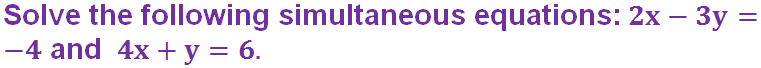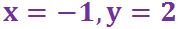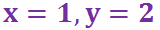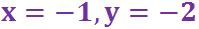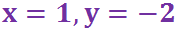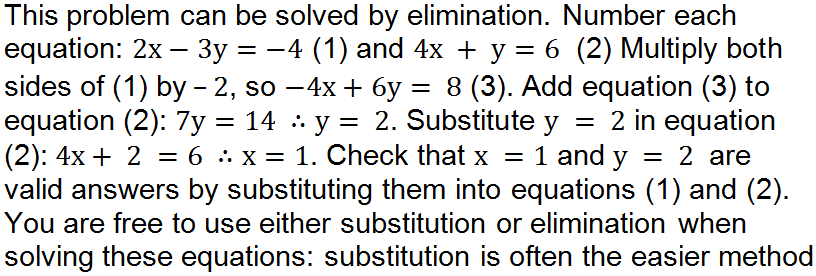Question 2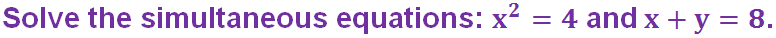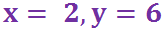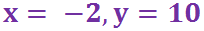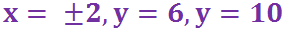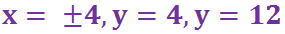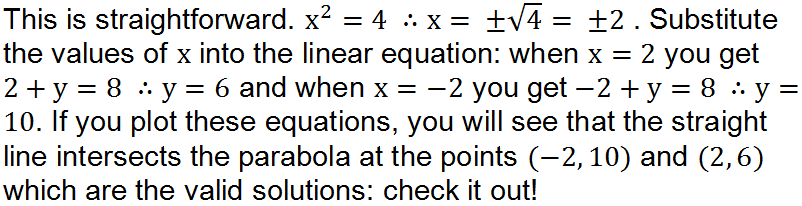Question 3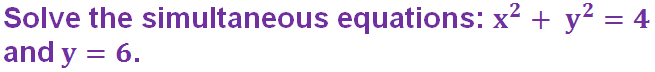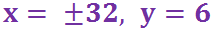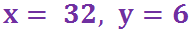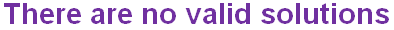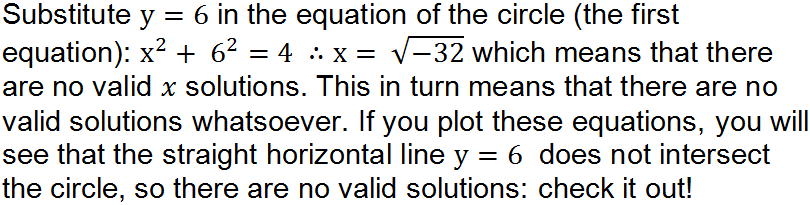Question 4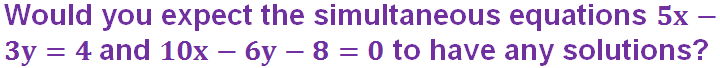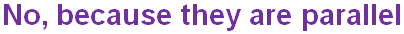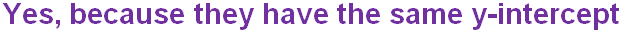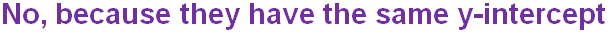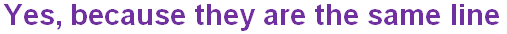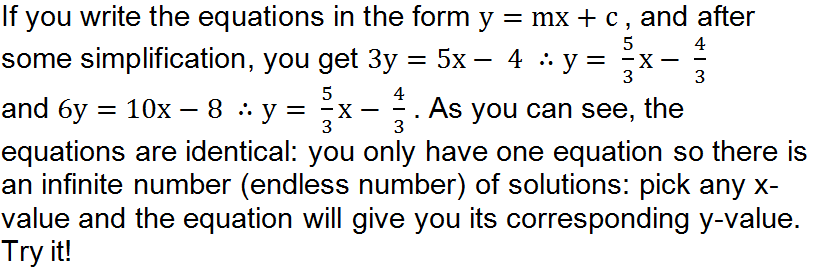Question 5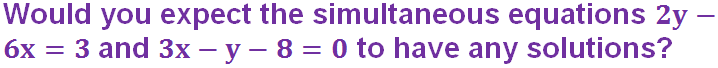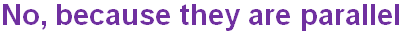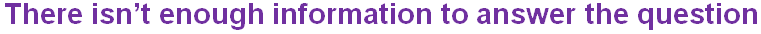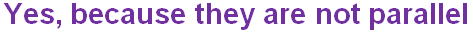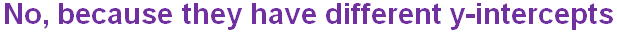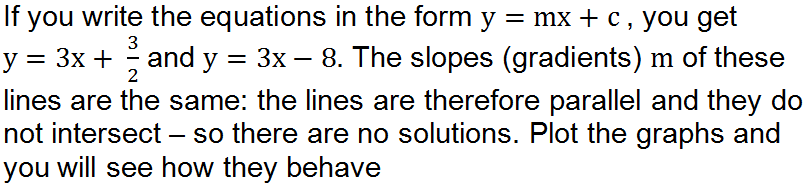Question 6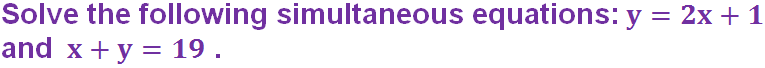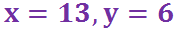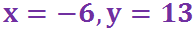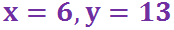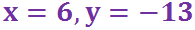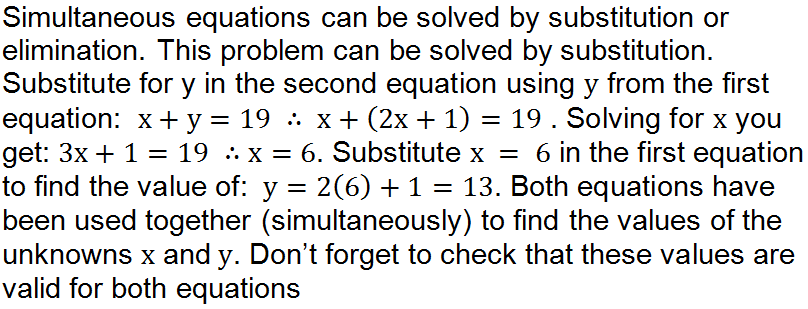Question 7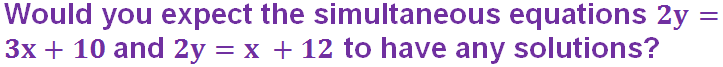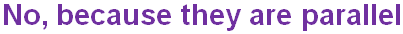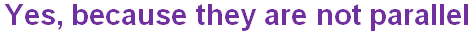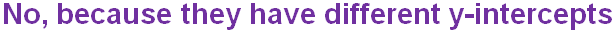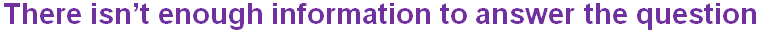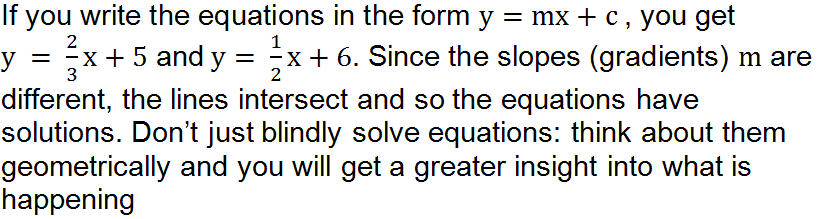Question 8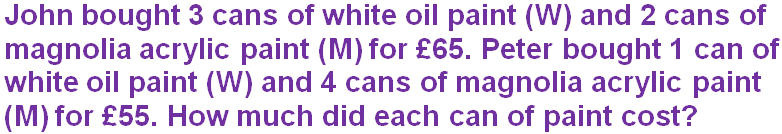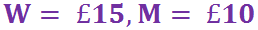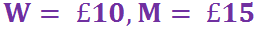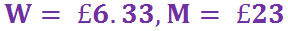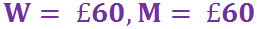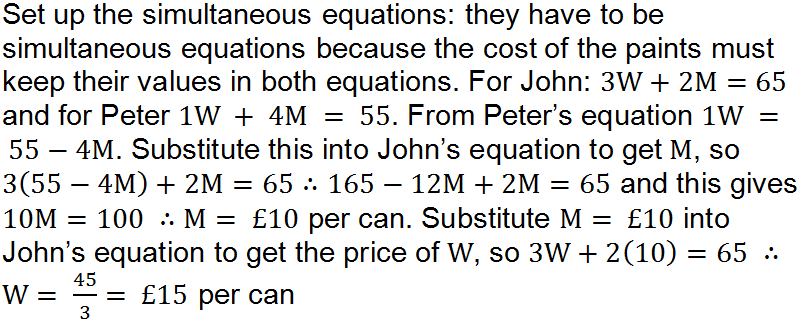Question 9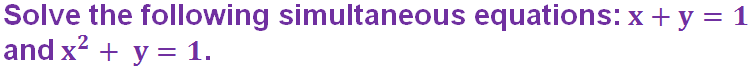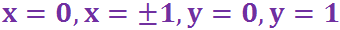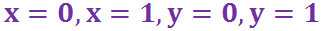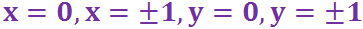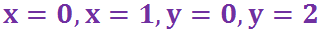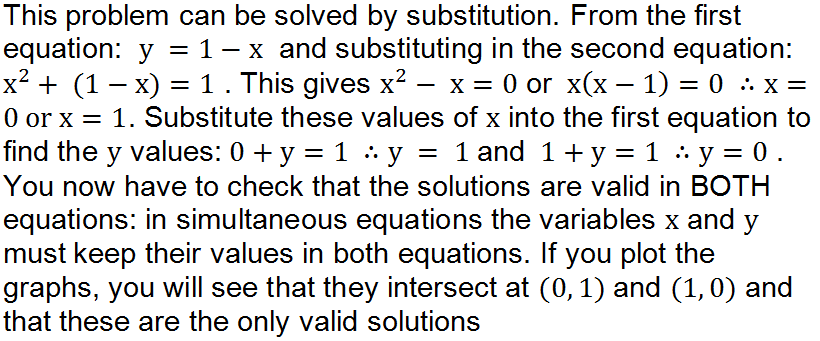Question 10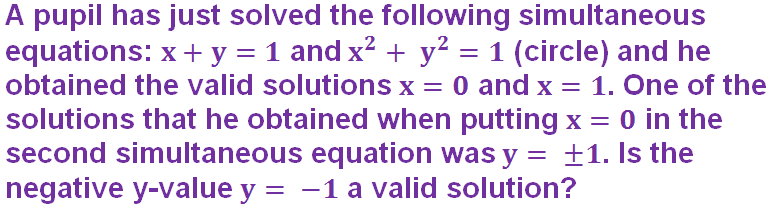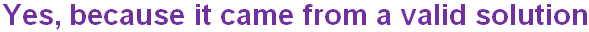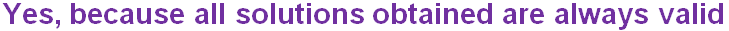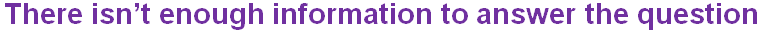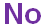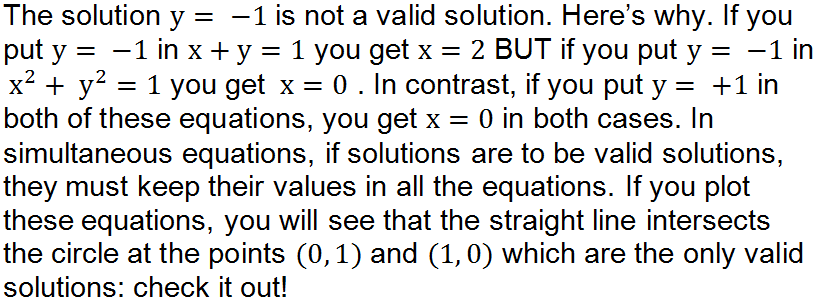Author:  Frank Evans